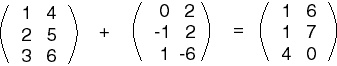No, they have the same dimensions, but corresponding elements are not equal.

If two matrices have the same number of rows and same number of columns, then the matrix sum can be computed:

If A is an MxN matrix, and B is also an MxN matrix, then their sum is an MxN matrix formed by adding corresponding elements of A and B.

Here is an example of this:Of course, in most practical situations the elements of the matrices are real numbers with decimal fractions, not the small integers often used in examples.

### QUESTION 7:

What 3x2 matrix could be added to a second 3x2 matrix without changing that second matrix?